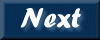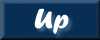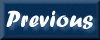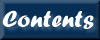Next: 5. Mosaics Up: XIMAGE User's Guide Previous: 3. Plot Images   Contents

Subsections

4. Source analysis

There are several XIMAGE commands to make a detailed analysis of the image. These include:

• BACKGROUND - estimate the background.

• EXCESS - list the image excesses using a sliding box.

• SEARCH - use the output list from excess to determine the good sources. It uses PSF and vignetting to estimate source significance. (Background+Excess+Search = Detect)

• DETECT - a sliding cell source detection algorithm, using the PSF and vignetting to estimate source significance and statistics.

• REMOVE - remove sources from an image.

• COUNTS - calculate the source counts in a given region

• SOSTA - calculate the source counting rates and statistics correcting for vignetting, point spread function and exposure

• PSF - generate the point spread function and compare it to the predicted psf.

All these commands but counts are very mission dependent and require the available calibration information.

4.1 Background

The BACKGROUND command estimates the background in the image. The method used divides the image into equal boxes to estimate the local background intensity. The event distribution in each box is checked for spatial uniformity and consistency with the expected statistical distribution (Poisson or Gaussian, depending on image type). If inconsistency between the observed and expected distribution is found the box is rejected. The distribution of the average background values in all boxes is then compared to a Gaussian distribution with mean equal to the mean of all the background values found. All boxes where the background level is more than three standard deviations away from the mean are rejected. If more than 80% of the boxes are rejected the background routine stops and an error message is generated. The value of the background intensity returned is the average of the values measured in all accepted boxes. A P(D) can be obtained using the background/npd=n/box=m/plot. The output is written to the file pd.qdp.

4.2 Sosta

The SOSTA command can be used to determine the count rate in a box region. SOSTA counts the number of events within a specified box, corrects those counts for vignetting, exposure and the point spread function to give the source intensity and its statistical significance. If the significance is less than 1.e-3 an upper limit is automatically calculated. The simplest way to use SOSTA is with the cursor, its default mode. SOSTA will then use a box that corresponds to 66% of the encircled energy function (EEF), and will use a background from a region surrounding the source, outside the 98% EEF boundary.

The background can be estimated using several different methods, specified by the user. These are:

• The background is estimated from a region within two boxes centered on the source using the option /INNER_RADIUS and /OUTER_RADIUS to specify the half-sizes of the two boxes. This is the default.

• The background is taken from any specified part of the image using /BOX_BACKGROUND;

• The background intensity can be given using the qualifier /BACKGROUND_LEVEL. In this case the background statistical error is assumed to be zero.

The uncertainty in the count rate returned by SOSTA is purely statistical i.e. does not include systematic errors. SOSTA will estimate the optimum box size to maximize the signal to noise ratio. This is given at the end of each run. By using the option /OPTIMIZE then SOSTA will go around a second time, and use the optimum box size to calculate the optimum source statistics.

The psf correction that is calculated can be weighted by an exposure map if the /EXPOWGT qualifier is given.

4.3 Counts

The COUNTS command sums the total number of counts specified either as a box with the cursor or within a region file. Complicated regions composed of any of the major shapes, such as box, circle, and polygon, may be input via a region file. The COUNTS command does not apply any correction to the total counts nor does it subtract the background.

4.4 Detect

The DETECT command locates point sources in the current image by means of a sliding-cell method. The average background intensity is estimated using the method described previously. The position and intensity of each detected source are calculated in a box whose size maximizes the signal-to-noise ratio. If the source is not pointlike the estimated count rate is in general inaccurate and likely to be under-estimated. A good estimate of the intensity of extended sources can be obtained with command COUNTS.

Corrections to the net counts are applied if the proper calibration information are available for that instrument. The corrections applied are dead times, vignetting and psf (the fraction of the source counts that fall outside the box where the net counts are estimated). Count rate errors include both statistical and systematic uncertainties added quadratically. To minimize the number of spurious sources detected the threshold used by detect is somewhat conservative. Consequently, some sources with intensity just above the image background can be missed. In order to allow detect to obtain a sufficiently good estimate of the background only images of size 128x128 pixels or larger should be used. Maximum accuracy is obtained running detect on full resolution images.

For crowded fields, or for fields with bright sources the qualifier /bright. This will make a weighted mean of each excess, and optimizing the psf. Consequently it can take much longer to run. For crowded images, e.g. ROSAT PSPC, it may be necessary to "tweak" the detection parameters to avoid false detections. The /thr_scal and /source_box qualifiers are particularly useful. The /thr_scal scales the threshold for excesses to be detected. This can be increased by modest amounts for ROSAT PSPC deep fields e.g. 1.2 or 1.3. The /source_box qualifier determines the size of the box used, in detector coordinates. For the ROSAT PSPC the default is 80, and it can be increased to 160 to reduce confusion.

The functionality of the detect command may also be accessed by executing the background, excess, and search commands in succession. Running the process in pieces allows for finer control of each step. For example, the background calculation may be tweaked, the output of each step can be written out and/or read in, and the image may be altered between steps.

4.5 Remove

Sources, or other features can be removed from an image using the command REMOVE. This can be done by:

• using the cursor to mark one or more box regions (/CURSOR)

• specifying a region file to remove (/REGIONFILE)

• entering the pixels coordinates of each box (/XMIN,/YMIN, etc)

• using the sources found in the previous run of DETECT or SEARCH.

The latter mode is useful for checking how good the detect run was. For a crowded region the sequence DETECT;REMOVE/DETECT;DETECT;REMOVE/DETECT can be used to clean the image of all sources. The removed area is filled with the average background value or by either an constant input value.

4.6 Extract

The extract command can be used to generate spectra and lightcurves from an input event file. The default is to use the region file generated by the box and circle commands. A new region file name is input using /region=file. Output spectra(/engfile=) and lightcurves(/qdpfile=) are in ASCII format. These products can be transform in FITS files and use within XSPEC and XRONOS. A direct way to get a spectrum and a lightcurve is using XSELECT.

The ASCII spectrum can be used within XIMAGE to calculate the energy dependence of the PSF for a given source with the command psf/engfile=. If the extracted spectrum can be used as a representative of a number of sources to calculate the energy-dependent PSF, set the ENGFILE keyword in the mdb equal to the extracted spectrum. Then the psf command will automatically use the value in ENGFILE.

The extract command is also useful to generate color-color diagrams for all the sources detected in the field of view with the detect or search command.

4.6.1 Color-Color Diagrams

The command extract/detect/plot_color will generate a color-color diagram for all sources detected with detect or search command. This is useful for identifying very soft or very hard sources in the field. The default channel boundaries can be changed using the /C1=/C2=/C3=/C4= qualifiers, or set ahead in the Mission database using the command chmdb (i.e. set the EXCHAN key as C1,C2,C3,C4). An output QDP format filename containing the results is written with a default of the eventfile.col, where eventfile is the input event filename. A new name is given with the qualifier colorfile=. The colors calculated correspond to the following ratios: (C4-C3)/(C3-C2) and (C2-C1)/(C3-C2). The qualifier cl3file= outputs the color-color results with additional columns, which contain the mean channel for each of the channel ranges.

4.7 PSF

Calculates differential and integral Point Spread Function, PSF. It also compares the observed and the expected PSF provided the latter is given.

The psf command accumulates all the counts within annuli of increasing radii. Each annulus increases by one pixel. Rebin the image to change the step size. The results are written to a ASCII file (QDP-compatible) and plotted. The differential PSF is given as a surface brightness (counts/s/sq arc min) and the integral as the Encircled Energy Function, EEF. The expected values for a pointlike source are also written to the QDP file and displayed. An accurate estimate of the image background is required, and is obtained using the background command. The cursor can be used to specify the source centroid, and the outer ring psf/cursor. The psf command can be used to extract the surface brightness profile of extended sources, for further analysis outside XIMAGE.

Typically, PSF depends on offaxis and energy, and the mathematical description can be provided via a subroutine. If no routine are available for a specific mission, the offaxis dependence of the PSF can be derived within XIMAGE with the /multpsffile= qualifier. This writes a PSF file which can be set as the PSFFILE key with the chmdb command, so detect and sosta use it for subsequent analysis. The file created using the qualifier multpsffile= can contain multiple columns representing the PSF at different offaxis angles.

If an exposure map is available, exposure map weighting can be included in the calculation with the /expowgt option. Each annulus is weighted by the total exposure in that annlulus, normalized to the maximum exposure contained within the outer radius of the largest annulus.

If the expected PSF is energy-dependent, the psf/engfile= allows to input a spectrum which will be used to calculate the observed PSF weighted for that spectrum. The extract/engfile= command generates the spectral file to input in psf/engfile=. If no spectrum is input, then the PSF is calculated at a single energy (1 keV is the default). The single energy is used to speed up detect and sosta. Currently, energy-dependent PSF routines are available within XIMAGE for ROSAT, ASCA, and BeppoSAX.

The following is an example of how to make a PSF for the ROSAT PSPC. This procedure is more complex because the PSF is energy dependent, so a spectrum must first be accumulated.

For ROSAT PSPC only:

read/rfits/xpix=5000/ypix=5000 rp500012.fits  ! make an image from the
! events file.
disp                                          ! display it
circle                                        ! define a source region
extract/engfile=eng_rosat.dat                 ! extract a spectrum
psf/engfile=eng_rosat.dat/cur                 ! use spectrum to calc psf

For all instruments (for non-PSPC first read in the image):

back/opt                                      ! optimum background
psf/cur/back=1.0e-03                          ! calculate the psfNext: 5. Mosaics Up: XIMAGE User's Guide Previous: 3. Plot Images   Contents